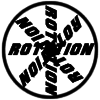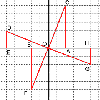#### You may also like### 8 Methods for Three by One

This problem in geometry has been solved in no less than EIGHT ways by a pair of students. How would you solve it? How many of their solutions can you follow? How are they the same or different? Which do you like best?### Rots and Refs

Follow hints using a little coordinate geometry, plane geometry and trig to see how matrices are used to work on transformations of the plane.### The Matrix

Investigate the transfomations of the plane given by the 2 by 2 matrices with entries taking all combinations of values 0. -1 and +1.

# Reflect Again

##### Age 16 to 18Challenge Level

The conditions suggested in the question give you two equations which you can solve to find $p'$ and $q'$. Use the same formulation to give $(p'',q'')$.

The problem 'The Matrix' explains how 2 by 2 matrices are used to give transformations of the plane and how you multiply the position vector of a point by the matrix to find the image of that point under the transformation.